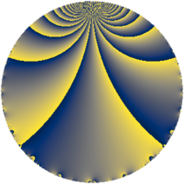# Properties

 Label 45.2.jLevel $45$ Weight $2$ Character orbit 45.j Rep. character $\chi_{45}(4,\cdot)$ Character field $\Q(\zeta_{6})$ Dimension $8$ Newform subspaces $1$ Sturm bound $12$ Trace bound $0$

# Related objects

## Defining parameters

 Level: $$N$$ $$=$$ $$45 = 3^{2} \cdot 5$$ Weight: $$k$$ $$=$$ $$2$$ Character orbit: $$[\chi]$$ $$=$$ 45.j (of order $$6$$ and degree $$2$$) Character conductor: $$\operatorname{cond}(\chi)$$ $$=$$ $$45$$ Character field: $$\Q(\zeta_{6})$$ Newform subspaces: $$1$$ Sturm bound: $$12$$ Trace bound: $$0$$

## Dimensions

The following table gives the dimensions of various subspaces of $$M_{2}(45, [\chi])$$.

Total New Old
Modular forms 16 16 0
Cusp forms 8 8 0
Eisenstein series 8 8 0

## Trace form

 $$8 q - 12 q^{6} + O(q^{10})$$ $$8 q - 12 q^{6} - 8 q^{10} - 12 q^{11} + 12 q^{15} + 4 q^{16} - 8 q^{19} + 12 q^{20} + 12 q^{21} + 12 q^{24} - 4 q^{25} + 24 q^{26} + 12 q^{29} - 12 q^{30} + 4 q^{31} - 4 q^{34} - 24 q^{35} - 12 q^{39} - 4 q^{40} - 24 q^{41} - 24 q^{44} - 16 q^{46} - 4 q^{49} + 24 q^{50} - 36 q^{51} + 24 q^{55} - 12 q^{56} + 24 q^{59} + 24 q^{60} - 8 q^{61} + 32 q^{64} + 60 q^{66} + 12 q^{69} + 12 q^{70} + 72 q^{71} - 12 q^{74} - 48 q^{75} - 12 q^{76} + 16 q^{79} - 48 q^{80} + 36 q^{81} - 36 q^{84} + 16 q^{85} - 36 q^{86} - 24 q^{89} - 36 q^{90} - 24 q^{91} + 20 q^{94} + 12 q^{95} - 60 q^{96} - 36 q^{99} + O(q^{100})$$

## Decomposition of $$S_{2}^{\mathrm{new}}(45, [\chi])$$ into newform subspaces

Label Dim $A$ Field CM Traces $q$-expansion
$a_{2}$ $a_{3}$ $a_{5}$ $a_{7}$
45.2.j.a $8$ $0.359$ $$\Q(\zeta_{24})$$ None $$0$$ $$0$$ $$0$$ $$0$$ $$q+\zeta_{24}^{6}q^{2}+(\zeta_{24}^{5}-\zeta_{24}^{7})q^{3}-\zeta_{24}^{3}q^{4}+\cdots$$